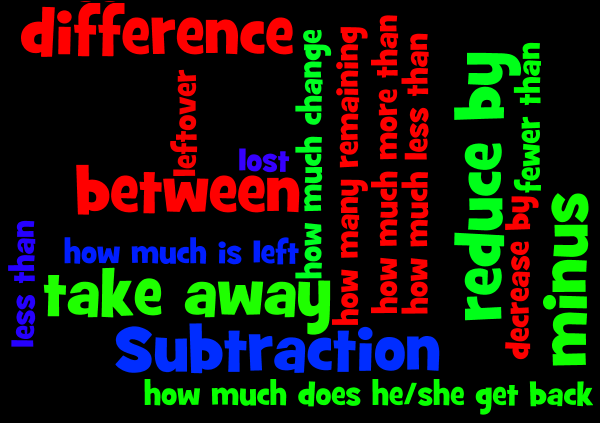## Friday

### Slamming Subtraction (Take Away)

Most students can pretty quickly recognise that when something is being taken away from a number, you use subtraction. It is the rare, skilful mathematician who also remembers to subtract when the situation involves finding the difference between two numbers, or how much more or less one number is compared to another. Most students mistakenly add: aaaaah!

That's a fair number of phrases that all mean subtraction, but if you read closely you'll notice that many sound VERY similar:## Subtraction facts

Before the big stuff, you need to make sure you know your basic number facts VERY well. Remember, subtraction is best friends with ..... addition! So, once you know your addition facts, you can just reverse these to learn subtraction.
For example:
you know                 8 + 3 = 11
and you know          3+ 8 = 11
so you also know:   11 - 3 = 8
and:                         11 - 8 = 3

Try building fact families to build on what you already know about addition to slam subtraction by clicking here

Here's some games for practice:
- Hit the Button: Click on Number Bonds in the bottom left corner, then click on:
- Up to 10: Missing Numbers
- Subtraction within 20
- Feed the aliens
- Dinosaur eggs (pick the subtraction option)
Pirate dash
- Deep-sea diving
- And the most challenging: Super Grid Speed Challenge 2

## Renaming in the vertical algorithm

What does renaming mean? How can I use one place value to help me subtract when I don't have enough ones, or tens, or hundreds, etc?

More on how renaming in subtraction actually works:
Subtracting: three digit numbers and basic regrouping: Subtracting multi-digit numbers can be a little confusing when the top digits are smaller than the bottom ones. Let's look at regrouping together. It will help!

Another example:

Practice renaming while bowling: (TIP: Make sure you do your working out on paper, DON'T try to do it just in your head on the computer because it's very hard and you need to start at the ones, not the hundreds!!!): Bowling through Renaming

## Renaming over a zero in a place value

Subtracting: Regrouping from zero: In Kindergarten you learned the importance of sharing, right? In math, when we lack value in a number to subtract with you borrow or regroup from a neighbour. Sometimes you borrow from the tens...but what if you have zero tens? Ha....let's watch this and learn!

Last one: Regrouping twice example: